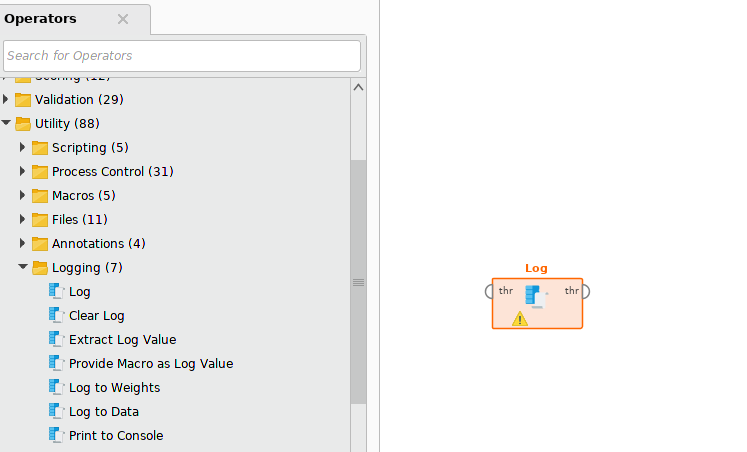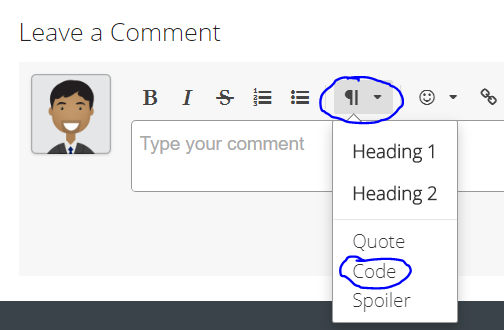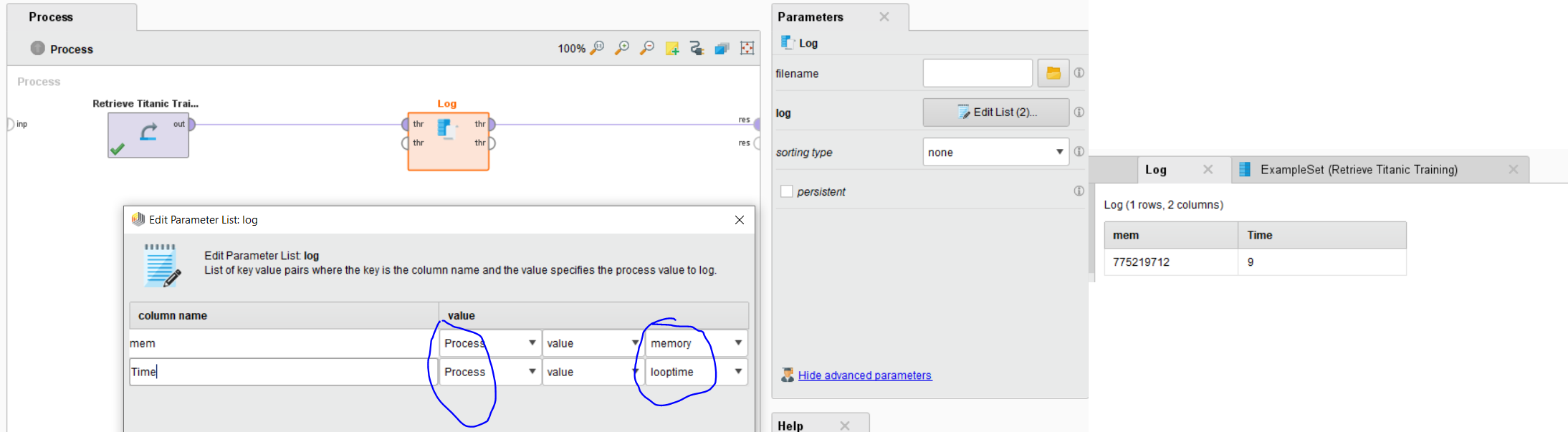# log operator

Member Posts: 12Contributor I
log operator memory value is in kilobytes? and i m using apriori .. what is the difference between apriori time and process time? Is  process indicates the whole model ?

• Administrator, Moderator, Employee, RMResearcher, Member Posts: 216RM Research
Sorry @mahajandiv ,
but I don't really understand your question.
The Log operator normally does not has an option to capture the memory or apriori time.

Do you use the operator shown in the picture below or some extension?Best,
David
• Member Posts: 12Contributor I
edited July 2019
yes i have use log operator.... and  one of its parameter log having edit list in which we add attributes with their values or parameters and then their is option of memory, time, loop time etc.. i want to send u the screenshot but its not posting that comment
• Moderator, Member Posts: 1,116Unicorn
Hello @mahajandiv

There are different processing times that can be logged using the log operator. To be clear, can you post, your XML code here? You can find XML code in XML window by clicking (View --> Show Panel --> XML). Copy the code from there and paste it here as shown in the image below.Regards,
Varun
https://www.varunmandalapu.com/

# Be Safe. Follow precautions and Maintain Social Distancing

• Member Posts: 12Contributor I
```<context>
<input/>
<output/>
<macros/>
</context>
<operator activated="true" class="process" compatibility="9.3.001" expanded="true" name="Process">
<parameter key="logverbosity" value="init"/>
<parameter key="random_seed" value="2001"/>
<parameter key="send_mail" value="never"/>
<parameter key="process_duration_for_mail" value="30"/>
<parameter key="encoding" value="SYSTEM"/>
<process expanded="true">
<operator activated="true" class="retrieve" compatibility="9.3.001" expanded="true" height="68" name="Retrieve 12traff" width="90" x="45" y="34">
<parameter key="repository_entry" value="12traff"/>
</operator>
<operator activated="true" class="discretize_by_frequency" compatibility="9.3.001" expanded="true" height="103" name="Discretize" width="90" x="179" y="85">
<parameter key="return_preprocessing_model" value="false"/>
<parameter key="create_view" value="false"/>
<parameter key="attribute_filter_type" value="all"/>
<parameter key="attribute" value=""/>
<parameter key="attributes" value="|avgMeasuredTime|avgSpeed|vehicleCount"/>
<parameter key="use_except_expression" value="false"/>
<parameter key="value_type" value="numeric"/>
<parameter key="use_value_type_exception" value="false"/>
<parameter key="except_value_type" value="real"/>
<parameter key="block_type" value="value_series"/>
<parameter key="use_block_type_exception" value="false"/>
<parameter key="except_block_type" value="value_series_end"/>
<parameter key="invert_selection" value="false"/>
<parameter key="include_special_attributes" value="false"/>
<parameter key="use_sqrt_of_examples" value="false"/>
<parameter key="number_of_bins" value="2"/>
<parameter key="range_name_type" value="long"/>
<parameter key="automatic_number_of_digits" value="true"/>
<parameter key="number_of_digits" value="-1"/>
</operator>
<operator activated="true" class="date_to_numerical" compatibility="9.3.001" expanded="true" height="82" name="Date to Numerical" width="90" x="313" y="136">
<parameter key="attribute_name" value="TIMESTAMP"/>
<parameter key="time_unit" value="millisecond"/>
<parameter key="millisecond_relative_to" value="second"/>
<parameter key="second_relative_to" value="minute"/>
<parameter key="minute_relative_to" value="hour"/>
<parameter key="hour_relative_to" value="day"/>
<parameter key="day_relative_to" value="month"/>
<parameter key="week_relative_to" value="year"/>
<parameter key="month_relative_to" value="year"/>
<parameter key="quarter_relative_to" value="year"/>
<parameter key="half_year_relative_to" value="year"/>
<parameter key="year_relative_to" value="era"/>
<parameter key="keep_old_attribute" value="false"/>
</operator>
<operator activated="true" class="numerical_to_binominal" compatibility="9.3.001" expanded="true" height="82" name="Numerical to Binominal" width="90" x="447" y="136">
<parameter key="attribute_filter_type" value="all"/>
<parameter key="attribute" value=""/>
<parameter key="attributes" value=""/>
<parameter key="use_except_expression" value="false"/>
<parameter key="value_type" value="numeric"/>
<parameter key="use_value_type_exception" value="false"/>
<parameter key="except_value_type" value="real"/>
<parameter key="block_type" value="value_series"/>
<parameter key="use_block_type_exception" value="false"/>
<parameter key="except_block_type" value="value_series_end"/>
<parameter key="invert_selection" value="false"/>
<parameter key="include_special_attributes" value="false"/>
<parameter key="min" value="0.0"/>
<parameter key="max" value="0.0"/>
</operator>
<operator activated="true" class="weka:W-Apriori" compatibility="7.3.000" expanded="true" height="68" name="W-Apriori" width="90" x="514" y="34">
<parameter key="N" value="1234567.0"/>
<parameter key="T" value="0.0"/>
<parameter key="C" value="0.2"/>
<parameter key="D" value="0.05"/>
<parameter key="U" value="1.0"/>
<parameter key="M" value="0.05"/>
<parameter key="S" value="-1.0"/>
<parameter key="I" value="true"/>
<parameter key="R" value="false"/>
<parameter key="V" value="false"/>
<parameter key="A" value="false"/>
<parameter key="c" value="-1.0"/>
</operator>
<operator activated="true" class="log" compatibility="9.3.001" expanded="true" height="82" name="Log (2)" width="90" x="648" y="34">
<parameter key="filename" value="C:\Users\computer\Documents\apriori.log"/>
<list key="log">
<parameter key="count" value="operator.Process.value.applycount"/>
<parameter key="Memory" value="operator.Process.value.memory"/>
<parameter key="process time" value="operator.Process.value.time"/>
<parameter key="Apriori time" value="operator.W-Apriori.value.looptime"/>
<parameter key="Support" value="operator.W-Apriori.parameter.M"/>
<parameter key="Confidence" value="operator.W-Apriori.parameter.C"/>
</list>
<parameter key="sorting_type" value="none"/>
<parameter key="sorting_k" value="100"/>
<parameter key="persistent" value="false"/>
</operator>
<connect from_op="Retrieve 12traff" from_port="output" to_op="Discretize" to_port="example set input"/>
<connect from_op="Discretize" from_port="example set output" to_op="Date to Numerical" to_port="example set input"/>
<connect from_op="Date to Numerical" from_port="example set output" to_op="Numerical to Binominal" to_port="example set input"/>
<connect from_op="Numerical to Binominal" from_port="example set output" to_op="W-Apriori" to_port="example set"/>
<connect from_op="W-Apriori" from_port="associator" to_op="Log (2)" to_port="through 1"/>
<connect from_op="Log (2)" from_port="through 1" to_port="result 1"/>
<portSpacing port="source_input 1" spacing="0"/>
<portSpacing port="sink_result 1" spacing="0"/>
<portSpacing port="sink_result 2" spacing="0"/>
</process>
</operator>
</process>
```

• Moderator, Member Posts: 1,116Unicorn
Hello @David_A

The log operator shows an option to capture Process Memory and several execution times like (loop time, CPU execution time etc). The only issue is there are no units for these outputs to understand. Below image is with process and results. Can you inform about this?